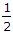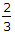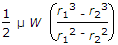# Online Mechanical Engineering Test - Theory of Machines Test 2Loading Test...

Instruction:

• This is a FREE online test. DO NOT pay money to anyone to attend this test.
• Total number of questions : 20.
• Time alloted : 30 minutes.
• Each question carry 1 mark, no negative marks.
• DO NOT refresh the page.
• All the best :-).

1.

In a coupling rod of a locomotive, each of the four pairs is a __________ pair.

A.
 slidingB.
 turningC.
 rollingD.
 screw2.

The factor which affects the critical speed of a shaft is

A.
 diameter of discB.
 span of shaftC.
 eccentricityD.
 all of these3.

The primary unbalanced force is maximum when the angle of inclination of the crank with the line of stroke is

A.
 0° and 90°B.
 0° and 180°C.
 90° and 180°D.
 180° and 360°4.

Which of the following is a higher pair?

A.
 Belt and pulleyB.
 Turning pairC.
 Screw pairD.
 Sliding pair5.

The distance between the centre of suspension and centre of percussion is equal to the equivalent length of a simple pendulum.

A.
 AgreeB.
 Disagree6.

The unbalanced primary forces in a reciprocating engine are

A.
 balanced completelyB.
 balanced partiallyC.
 balanced by secondary forcesD.
 not balanced7.

When a point at the end of a link moves with constant angular velocity, its acceleration will have

A.
 radial component onlyB.
 tangential component onlyC.
 coriolis component onlyD.
 radial and tangential components both8.

A watt's governor can work satisfactorily at speeds from

A.
 60 to 80 r.p.m.B.
 80 to 100 r.p.m.C.
 100 to 200 r.p.m.D.
 200 to 300 r.p.m.9.

A body of weight W is required to move up the rough inclined plane whose angle of inclination with the horizontal is α. The effort applied parallel to the plane is given by (where μ = tan φ = Coefficient of friction between the plane and the body)

A.
 P = W tan αB.
 P = W tan (α + φ)C.
 P = W (sin α + μ cos α)D.
 P = W (cos α + μ sin α)10.

The lower pairs are __________ pairs.

A.
 self-closedB.
 force-closed11.

The frictional torque transmitted by a disc or plate clutch is same as that of

A.
 flat pivot bearingB.
 flat collar bearingC.
 conical pivot bearingD.
 truncated conical pivot bearing12.

The maximum gyroscopic couple acting on the ship tends to shear the holding down bolts.

A.
 CorrectB.
 Incorrect13.

For the isochronous Porter governor, the controlling force curve is a straight line passing through the origin.

A.
 CorrectB.
 Incorrect14.

When no external force acts on a body, after giving it an initial displacement, the body is said to have free vibrations.

A.
 TrueB.
 False15.

A shaft of mass (mc)and stiffness (s) is fixed at one end and carries a mass (m) at the other end. The natural frequency of its transverse vibration is same as for longitudinal vibration.

A.
 YesB.
 No16.

A sliding pair has a completely constrained motion.

A.
 YesB.
 No17.

When the connection between the elements forming a pair is such that the constrained motion is not completed by itself, but by some other means, the motion is said to be a completely constrained motion,

A.
 YesB.
 No18.

A pair is said to be a kinematic pair, if the relative motion between them is completely or successfully constrained.

A.
 CorrectB.
 Incorrect19.

When a point moves along a straight line, its acceleration will have

A.
 radial component onlyB.
 tangential component onlyC.
 coriolis component onlyD.
 radial and tangential components both20.

The frictional torque transmitted in a flat collar bearing, considering uniform pressure, is (where r1 and r2 = External and internal radii of collar respectively)

A.μ W (r1 + r2)B.μ W (r1 + r2)C.D.